### Home > CC1 > Chapter 7 > Lesson 7.3.4 > Problem7-128

7-128.
1. Use a Giant One to change each of the following fractions to a number with a denominator of 100. Then write each portion as a percent. Homework Help ✎

1.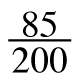2.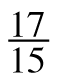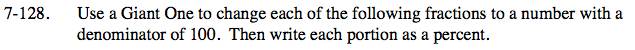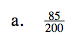200 times what is 100? This number will be the denominator, or the bottom of the fraction, in your Giant One.
Remember, in a Giant One the numerator, or the number on top, and the denominator will be the same so that the fraction is equal to one.

$\text{Here\ your\ Giant\ One\ should\ be\: \frac{0.5}{0.5}.\ }$

$\text{Can\ you\ multiply\\ \frac{85}{200}\ and\ \frac{0.5}{0.5}\\ to\ get\ a\ }$

$\text{new\ fraction\\ with\ a\ denominator\ of\ 100?}$

$\frac{85}{200}=\frac{42.5}{100}$

Were you able to find the percent equivalent? Refer to the Math Notes excerpt below for some help.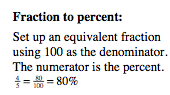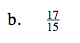15 times what will be equal to 100?
Try using division to find your Giant One's denominator and numerator.

$\text{The\ fraction\ will\ be\ equivalent\ to\ \frac{113.\overline{3}}{100}.}$

What would this be as a percent?